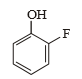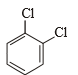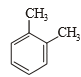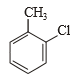Which of the following would have a permanent dipole moment ?

(a) BF3

(b) SiF4

(c) SF4

(d) XeF4

Concept Questions :-

Polarity
High Yielding Test Series + Question Bank - NEET 2020

Difficulty Level:

In the case of alkali metals, the covalent character decreases in the order

(a) MCl > MI > MBr > MF

(b) MF > MCl >  MBr > M

(c)MF > MCl > MI > MBr

(d) MI > MBr > MCl > MF

Concept Questions :-

Polarity
High Yielding Test Series + Question Bank - NEET 2020

Difficulty Level:

Which of the following is correct order of dipole moment of the following ?(1) I >  II > III

(2) I = II = III

(3) III > II > I

(4) I > III > II

Concept Questions :-

Polarity
High Yielding Test Series + Question Bank - NEET 2020

Difficulty Level:

which is the incorrect about bond angles ?

1. NH3 > NF3

2. NF3 <  PF3

3. NH3 > PH3

4. NH3 > H2O

Concept Questions :-

Polarity
High Yielding Test Series + Question Bank - NEET 2020

Difficulty Level:

In which case observed dipole moment is greater than theoretical dipole moment?

1.2.3.4.Concept Questions :-

Polarity
High Yielding Test Series + Question Bank - NEET 2020

Difficulty Level:

Which of the following molecules has the maximum dipole moment?

(a) CO2

(b) CH4

(c) NH3

(d) NF3

Concept Questions :-

Polarity
High Yielding Test Series + Question Bank - NEET 2020

Difficulty Level:

Dipole-induced dipole interactions are present in which of the following pairs?

(a) H2O and alcohol                        (b) Cl2 and CCl4

(c) HCl and He atoms                      (d) SiF4 and He atoms

Concept Questions :-

Polarity
High Yielding Test Series + Question Bank - NEET 2020

Difficulty Level:

Which of the following is a polar molecule?

(a) BF3

(b) SF4

(c) SiF4

(d) XeF4

Concept Questions :-

Polarity
High Yielding Test Series + Question Bank - NEET 2020

Difficulty Level:

Molecule having non-polar as well as polar bonds but the molecule as a whole is polar:

(a)

(b) ${\mathrm{N}}_{2}{\mathrm{O}}_{4}$

(c) ${\mathrm{Si}}_{2}{\mathrm{H}}_{6}$

(d) ${\mathrm{I}}_{2}{\mathrm{Cl}}_{6}$

Concept Questions :-

Polarity
High Yielding Test Series + Question Bank - NEET 2020

Difficulty Level:

Which of the following species has polar and non-polar bonds but molecule as a whole is non-polar?

(a) ${\mathrm{S}}_{2}{\mathrm{O}}_{3}^{2-}$                                              (b) $\left(\mathrm{SCN}{\right)}_{2}$

(c) ${\mathrm{Be}}_{2}{\mathrm{Cl}}_{4}$                                              (d) ${\mathrm{Si}}_{2}{\mathrm{H}}_{6}$

Concept Questions :-

Polarity